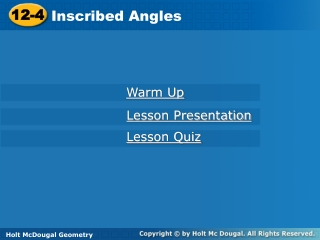DownloadDownload Presentation12-4

# 12-4

Télécharger la présentation## 12-4

- - - - - - - - - - - - - - - - - - - - - - - - - - - E N D - - - - - - - - - - - - - - - - - - - - - - - - - - -
##### Presentation Transcript

1. 12-4 Inscribed Angles Warm Up Lesson Presentation Lesson Quiz Holt McDougal Geometry Holt Geometry

2. Warm Up Find each value. 1.mBCA 2.t Solve for x. 3.58 – x = 4 (x + 7) 4. 2 (x – 8) = 8 63.5° 116.5° 6 12

3. Objectives Find the measure of an inscribed angle. Use inscribed angles and their properties to solve problems.

4. Vocabulary inscribed angle intercepted arc subtend

5. String art often begins with pins or nails that are placed around the circumference of a circle. A long piece of string is then wound from one nail to another. The resulting pattern may include hundreds of inscribed angles.

6. An inscribed angleis an angle whose vertex is on a circle and whose sides contain chords of the circle. An intercepted arcconsists of endpoints that lie on the sides of an inscribed angle and all the points of the circle between them. A chord or arc subtendsan angle if its endpoints lie on the sides of the angle.

7. Substitute 118 for mPU. Example 1A: Finding Measures of Arcs and Inscribed Angles Find each measure. mPRU Inscribed  Thm.

8. mSP Example 1B: Finding Measures of Arcs and Inscribed Angles Find each measure. Inscribed  Thm. Substitute 27 for m SRP. Multiply both sides by 2.

9. Check It Out! Example 1a Find each measure. Inscribed  Thm. Substitute 135 for m ABC. Multiply both sides by 2.

10. Substitute 76 for mDE. Check It Out! Example 1b Find each measure. mDAE Inscribed  Thm.

11. Example 2: Hobby Application An art student turns in an abstract design for his art project. Find mDFA. mDFA = mDCF + mCDF Ext  Thm. Inscribed  Thm. Substitute. Simplify. = 115°

12. Find mABD and mBC in the string art. Check It Out! Example 2 Inscribed  Thm. Substitute. =43 Inscribed  Thm. Substitute.

13. Example 3A: Finding Angle Measures in Inscribed Triangles Find a. 5a + 20 = 90 Substitute 5a + 20 for mWZY. 5a = 70 Subtract 20 from both sides. a = 14 Divide both sides by 5.

14. mLJM = mLKMmLJM and mLKM both intercept LM. Example 3B: Finding Angle Measures in Inscribed Triangles Find mLJM. 5b – 7 = 3b Substitute the given values. 2b – 7 = 0 Subtract 3b from both sides. 2b = 7 Add 7 to both sides. b = 3.5 Divide both sides by 2.

15. Check It Out! Example 3a Find z. 8z – 6 = 90 Substitute. 8z = 96 Add 6 to both sides. z = 12 Divide both sides by 8.

16. mEDF = mEGFmEGF and mEDF both intercept EF. Check It Out! Example 3b Find mEDF. 2x + 3 = 75 – 2x Substitute the given values. 4x = 72 Add 2x and subtract 3 from both sides. x = 18 Divide both sides by 4. mEDF= 2(18) + 3 = 39°

17. Example 4: Finding Angle Measures in Inscribed Quadrilaterals Find the angle measures of GHJK. Step 1 Find the value of b.

18. Example 4 Continued Step 2 Find the measure of each angle.

19. Check It Out! Example 4 Find the angle measures of JKLM. Step 1 Find the value of b. mM + mK = 180 JKLM is inscribed in a . 4x – 13 + 33 + 6x = 180 Substitute the given values. 10x + 20 = 180 Simplify. 10x = 160 Subtract 20 from both sides. x = 16 Divide both sides by 10.

20. Check It Out! Example 4 Continued Find the angle measures of JKLM. Step 2 Find the measure of each angle. mM = 4(16) – 13 = 51 mK = 33 + 6(16) = 129 mJ = 360 – 252 = 108

21. Lesson Quiz: Part I Find each measure. 1. RUS 2. a 25° 3

22. Lesson Quiz: Part II 3. A manufacturer designs a circular ornament with lines of glitter as shown. Find mKJN. 130° 4. Find the angle measures of ABCD. mA = 95° mB = 85° mC = 85° mD = 95°